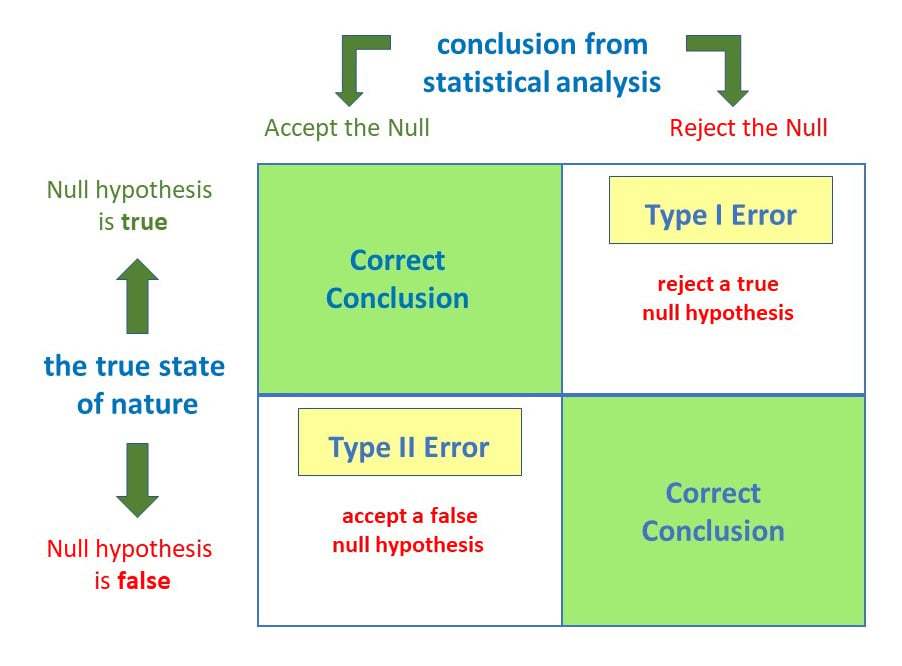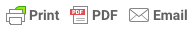# What are Type I and Type II Errors?

### What are Type I and Type II Errors?

By Saul McLeod, published July 04, 2019

A statistically significant result cannot prove that a research hypothesis is correct (as this implies 100% certainty). Because a p-value is based on probabilities, there is always a chance of making an incorrect conclusion regarding accepting or rejecting the null hypothesis (H0).

Anytime we make a decision using statistics there are four possible outcomes, with two representing correct decisions and two representing errors.The chances of committing these two types of errors are inversely proportional: that is, decreasing type I error rate increases type II error rate, and vice versa.

###### How does a Type 1 error occur?

A type 1 error is also known as a false positive and occurs when a researcher incorrectly rejects a true null hypothesis. This means that your report that your findings are significant when in fact they have occurred by chance.

The probability of making a type I error is represented by your alpha level (α), which is the p-value below which you reject the null hypothesis. A p-value of 0.05 indicates that you are willing to accept a 5% chance that you are wrong when you reject the null hypothesis.

You can reduce your risk of committing a type I error by using a lower value for p. For example, a p-value of 0.01 would mean there is a 1% chance of committing a Type I error.

However, using a lower value for alpha means that you will be less likely to detect a true difference if one really exists (thus risking a type II error).

###### How does a Type II error occur?

A type II error is also known as a false negative and occurs when a researcher fails to reject a null hypothesis which is really false. Here a researcher concludes there is not a significant effect, when actually there really is.

The probability of making a type II error is called Beta (β), and this is related to the power of the statistical test (power = 1- β). You can decrease your risk of committing a type II error by ensuring your test has enough power.

You can do this by ensuring your sample size is large enough to detect a practical difference when one truly exists.

###### Why are Type I and Type II Errors Important?

The consequences of making a type I error mean that changes or interventions are made which are unnecessary, and thus waste time, resources, etc.

Type II errors typically lead to the preservation of the status quo (i.e. interventions remain the same) when change is needed.